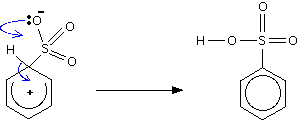# The Sulfonation of Benzene

$$\newcommand{\vecs}{\overset { \rightharpoonup} {\mathbf{#1}} }$$ $$\newcommand{\vecd}{\overset{-\!-\!\rightharpoonup}{\vphantom{a}\smash {#1}}}$$$$\newcommand{\id}{\mathrm{id}}$$ $$\newcommand{\Span}{\mathrm{span}}$$ $$\newcommand{\kernel}{\mathrm{null}\,}$$ $$\newcommand{\range}{\mathrm{range}\,}$$ $$\newcommand{\RealPart}{\mathrm{Re}}$$ $$\newcommand{\ImaginaryPart}{\mathrm{Im}}$$ $$\newcommand{\Argument}{\mathrm{Arg}}$$ $$\newcommand{\norm}{\| #1 \|}$$ $$\newcommand{\inner}{\langle #1, #2 \rangle}$$ $$\newcommand{\Span}{\mathrm{span}}$$ $$\newcommand{\id}{\mathrm{id}}$$ $$\newcommand{\Span}{\mathrm{span}}$$ $$\newcommand{\kernel}{\mathrm{null}\,}$$ $$\newcommand{\range}{\mathrm{range}\,}$$ $$\newcommand{\RealPart}{\mathrm{Re}}$$ $$\newcommand{\ImaginaryPart}{\mathrm{Im}}$$ $$\newcommand{\Argument}{\mathrm{Arg}}$$ $$\newcommand{\norm}{\| #1 \|}$$ $$\newcommand{\inner}{\langle #1, #2 \rangle}$$ $$\newcommand{\Span}{\mathrm{span}}$$$$\newcommand{\AA}{\unicode[.8,0]{x212B}}$$

This page gives you the facts and a simple, uncluttered mechanism for the electrophilic substitution reaction between benzene and sulfuric acid (or sulfur trioxide).

## The electrophilic substitution reaction between benzene and sulfuric acid

There are two equivalent ways of sulfonating benzene:

• Heat benzene under reflux with concentrated sulfuric acid for several hours.
• Warm benzene under reflux at 40°C with fuming sulfuric acid for 20 to 30 minutes.

$C_6H_6 + H_2SO_4 \rightarrow C_6H_5SO_3H + H_2O$

Or:The product is benzenesulfonic acid. The electrophile is actually sulfur trioxide, SO3, and you may find the equation for the sulfonation reaction written:## The formation of the electrophile

The sulfur trioxide electrophile arises in one of two ways depending on which sort of acid you are using. Concentrated sulfuric acid contains traces of SO3 due to slight dissociation of the acid.

$H_2SO_4 \rightleftharpoons H_2O + SO_3$

Fuming sulfuric acid, $$H_2S_2O_7$$, can be thought of as a solution of $$SO_3$$ i n sulfuric acid - and so is a much richer source of the SO3. Sulfur trioxide is an electrophile because it is a highly polar molecule with a fair amount of positive charge on the sulfur atom. It is this which is attracted to the ring electrons.

## The electrophilic substitution mechanism

Stage oneStage twoThe second stage of the reaction involves a transfer of the hydrogen from the ring to the negative oxygen.

## Contributors

This page titled The Sulfonation of Benzene is shared under a CC BY-NC 4.0 license and was authored, remixed, and/or curated by Jim Clark.